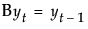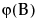An AutoRegressive Integrated Moving Average (ARIMA) model predicts future values of a time series by a linear combination of its past values and a series of errors (also known as random shocks or innovations). The ARIMA command performs a maximum likelihood fit of the specified ARIMA model to the time series.
t is the time index
B is the backshift operator defined asμ is the intercept or mean term.where the constant estimate δ is given by the relation.
 1 The Autoregressive Order is the order (p) of the polynomialoperator.
 2 The Differencing Order is the order (d) of the differencing operator.
 3 The Moving Average Order is the order (q) of the differencing operator.
 4 An ARIMA model is commonly denoted ARIMA(p,d,q). If any of p,d, or q are zero, the corresponding letters are often dropped. For example, if p and d are zero, then model would be denoted MA(q).
The Confidence Intervals box allows you to set the confidence level between 0 and 1 for the forecast confidence bands. The Intercept check box determines whether the intercept term μ will be part of the model. If the Constrain fit check box is checked, the fitting procedure will constrain the autoregressive parameters to always remain within the stable region and the moving average parameters within the invertible region. You might want to uncheck this box if the fitter is having difficulty finding the true optimum or if you want to speed up the fit. You can check the Model Summary table to see if the resulting fitted model is stable and invertible.